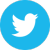# Courses Mathematical Physics

Top: Science: Physics: Mathematical Physics: Courses

• Spinors in Physics and Geometry - A fourth year math/physics course at UIUC with physics online lecture notes.
• Mathematical Tools for Physics - A text on the mathematics needed for upper level undergraduate physics physics courses by James Nearing, University of Miami (PDF).
• Methods of Mathematical Physics I - A set of lecture notes for this one semester course.
• Linear Methods of Applied Mathematics - This is a WWW textbook written by Evans M. Harrell II and James V. Herod, both of Georgia Tech. It is suitable for a first course on partial differential equations, Fourier series and special functions, and integral equations. Students are expected to h
• Preparation for Gauge Theory - Class lecture notes at a graduate level on mathematical physics the mathematical background needed to understand classical mathematical physics gauge theory.
• Mathematical Methods in Physics - Covers Conformal Field Theory, Modular Functor, Topological Quantum Field Theory and Frobenius Algebras,

MySQL - Cache Direct